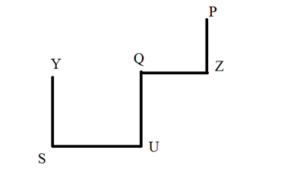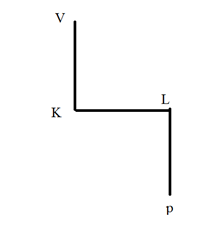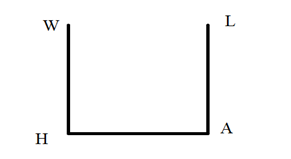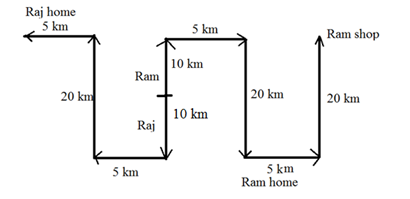# Crack SBI Clerk 2018 – Logical Reasoning (Directions and Coding Decoding) Day-31

## Crack SBI Clerk 2018 – Logical Reasoning (Directions and Coding Decoding) Day-31:

Dear Readers, Reasoning Section play a vital role in Banking and all other competitive exams. This year 2018 was started with three wonderful opportunities; Most Awaited SBI Clerk 2018. To enrich your preparation here we have providing new series of Practice Questions on Reasoning Ability – Directions and Coding Decoding. Candidates those who are going to appear in Bank Exams 2018 can practice these questions daily and make your preparation effective.

[WpProQuiz 1517]

Click “Start Quiz” to attend these Questions and view Solutions

## Daily Practice Test Schedule | Good Luck

 Topic Daily Publishing Time Daily News Papers & Editorials 8.00 AM Current Affairs Quiz 9.00 AM Logical Reasoning 10.00 AM Quantitative Aptitude “20-20” 11.00 AM Vocabulary (Based on The Hindu) 12.00 PM Static GK Quiz 1.00 PM English Language “20-20” 2.00 PM Banking Awareness Quiz 3.00 PM Reasoning Puzzles & Seating 4.00 PM Daily Current Affairs Updates 5.00 PM Data Interpretation / Application Sums (Topic Wise) 6.00 PM Reasoning Ability “20-20” 7.00 PM English Language (New Pattern Questions) 8.00 PM General / Financial Awareness Quiz 9.00 PM

Direction (1-3) Read the following information carefully and answers the questions given below

P % Q means P is South of Q

P \$ Q means Q is west of P

P @ Q means P is west of Q

P& Q means P is north of Q

1) In Which of the following expression Z is East of Y . If all point have equal distance ?

a) Z%[email protected]%P\$Y\$U

b) [email protected]@Z%P\$Y\$U

c) P&Z\$Q&U\$S%Y

d) Cannot be determined

e) None of these

2) What is the direction of V with Respect to P in the given expression

[email protected],K%V,L\$K,L&P

A) North east

b) South west

c) South east

d) Nort west

e) None of these

3) In all point have equal distance then which of the following symbols will come in place of question mark (?) to make the Expression T is North of A is definitely true ?

[email protected]&L ? W&[email protected]

a) %

b) @

c) &

d) \$

e) None of these

Direction (4-5) Read the following information carefully and answers the questions given below

If Ram and Raj are two friends . They start walking from same point but in opposite direction . Ram walks towards north . After Walking 10 km ram takes a right turn and walks for 5km then turns 90 degree clockwise direction and walks 20 km to reach his home and takes a left turn and walks 5 km . Raj also follows the same condition as Ram .

4) If Ram takes another left turn and walks 20 km to reach his shop. Then find the shortest distance between Ram’s shop to Ram’s home?

a) 912

b) 415

c) 517

d) 823

e) None of these

5) What is the direction of Raj’s home with respect to the starting point?

a) North west

b) south west

c) South east

d) East

e) None of these

6) If + “stands for division”

^  “stands for multiplication”

< “stands for subtraction”

−  “stands for equal to”

× “stands for greater than”

=  “stands for less  than”  which one of the following equations is correct ?

a) 14+7>3=6+3>2

b) 6+3>8=4+2<1

c) 4>6+2×32+4<1

d) 8<4+2=6>3

e) None of these

7) If 100 * 50 \$ 5 # 10 * 1000 @ 10=0,then which of the following mathematical operations are correct?

a) * means +, \$ means -, # means × and @ means ÷

b) * means -, \$ means +, # means × and @ means ÷

c) * means -, \$ means +, # means÷ and @ means ×

d) * means +, \$ means -, # means ÷ and @ means ×

e) None of these

8) If -‟ stands for division”

+ ‟ stands for multiplication”

÷ ‟ stands for subtraction” and

× ‟ stands for addition” which one of the following equations is correct ?

a) 6÷20 ×12+7-1=70

b) 6+20 -12×7-1=62

c) 6-20 ÷12×7+1=57

d) 6+20-12÷7-1=38

e) None of these

9) If 200×100+300×200-10÷2+40=0,which of the following mathematical operations are correct?

a) + means −, − means ×, × means ÷, and ÷ means +

b) + means −, − means ÷, × means +, and ÷ means ×

c) + means ×, − means −, × means ÷, and ÷ means +

d) + means ÷, − means +, × means − , and − means ×

e) None of these

10) If 50 + 150 ÷ 5+ 20 × 100 -10 = 10, then which of the following mathematical operations are correct?

a) × means ÷, + means -, ÷ means × and –means +

b) × means -, + means ÷, ÷ means + and– means ×

c) × means +, + means -, ÷ means ÷ and -means ×

d) × means +, + means -, ÷ means × and -means ÷

e) None of these

Direction (1-3)Direction (4-5)6) Answer d) 8 – 4 ÷ 2 < 6+3 6 < 9

7) Answer b) = 100 – 50 + 5 × 10 – 1000 ÷ 10 = 100 – 50 + 5 × 10 – 100 = 100 – 50 +50 – 100 = 0

8) Answer a) = 6-20+12× 7 ÷ 1 =6-20+12×7 =6-20+84=70

9) Answer b) = 200 + 100 – 300 + 200 ÷ 10 × 2 – 40 =300-300+20× 2 – 40 =0+40-40 = 0

10) Answer d) = 50-150×  5 – 20+100÷ 10 =50 – 30 – 20+10 =10